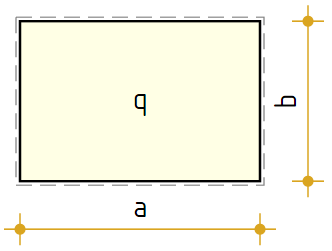### Elastic Analysis of Rectangular Slab

Dimensions in plan - a =   m , b =   m

 Slab thickness - t =   m Distributed load - q =   kNm2 Elastic modulus - E =   MPa Poisson`s ratio - ν =   Number of iterations - N =